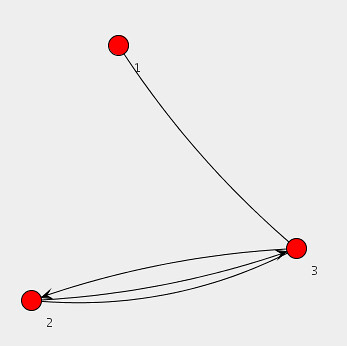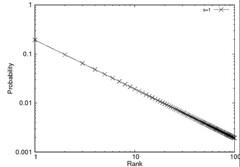# Java Universal Network/Graph Framework

Recently, I’m primarily concerned with large-scale graph data processing. Occasionally, the visualization of graph can be a good way for us to observe some properties from graph data sets. Today, I’m going to introduce a graph framework, called Java Universal Network/Graph Framework (Jung). Jung provides data structures for graph, a programming interface familiar with graph features, some fundamental graph algorithms (e.g., minimum spanning tree, depth-first search, breath-first search, and dijkstra algorithm), and even visualization methods. Especially, I’m interested in its visualization methods.

The following java source shows the programming interface of Jung. In more detail, this program make a graph, add three vertices to the graph, and connect vertices. This source code is brought from Jung tutorial. As you can see, Jung’s APIs are very easy.

```  // Make a graph by a SparseMultigraph instance.
Graph&lt;Integer, String&gt; g = new SparseMultigraph&lt;Integer, String&gt;();
g.addEdge(&quot;Edge-A&quot;, 1,3); // Added an edge to connect between 1 and 3 vertices.
g.addEdge(&quot;Edge-P&quot;, 2,3); // A parallel edge

// Make some objects for graph layout and visualization.
Layout&lt;Integer, String&gt; layout = new KKLayout&lt;Integer, String&gt;(g);
BasicVisualizationServer&lt;Integer, String&gt; vv =
new BasicVisualizationServer&lt;Integer, String&gt;(layout);
vv.setPreferredSize(new Dimension(800,800));

// It determine how each vertex with its value is represented in a diagram.
ToStringLabeller&lt;Integer&gt; vertexPaint = new ToStringLabeller&lt;Integer&gt;() {
public String transform(Integer i) {
return &quot;&quot;+i;
}
};

vv.getRenderContext().setVertexLabelTransformer(vertexPaint);

JFrame frame = new JFrame(&quot;Simple Graph View&quot;);
frame.setDefaultCloseOperation(JFrame.EXIT_ON_CLOSE);
frame.pack();
frame.setVisible(true);
```

Some APIs of the Jung are based on generic programming, so you can use easily vertices or edges to contains user-defined data. If you want more detail information, visit http://jung.sourceforge.net.

The above source code shows the following diagram.# Zipf Distribution Random Generator in Java

When I carry out some experiments, I usually make synthetic data sets generated by  some probability distributions.  Especially, Zipf distribution is frequently used for a synthetic data set. Zipf distribution is  one of the discrete power law probability distributions. You can get detail information from Zipf’s law in Wikipedia. Anyway, I attached my own java class for zip distribution. Below graphs are generated by my own java code and the gnuplot.

```import java.util.Random;

public class ZipfGenerator {
private Random rnd = new Random(System.currentTimeMillis());
private int size;
private double skew;
private double bottom = 0;

public ZipfGenerator(int size, double skew) {
this.size = size;
this.skew = skew;

for(int i=1;i < size; i++) {
this.bottom += (1/Math.pow(i, this.skew));
}
}

// the next() method returns an random rank id.
// The frequency of returned rank ids are follows Zipf distribution.
public int next() {
int rank;
double friquency = 0;
double dice;

rank = rnd.nextInt(size);
friquency = (1.0d / Math.pow(rank, this.skew)) / this.bottom;
dice = rnd.nextDouble();

while(!(dice &lt; friquency)) {
rank = rnd.nextInt(size);
friquency = (1.0d / Math.pow(rank, this.skew)) / this.bottom;
dice = rnd.nextDouble();
}

return rank;
}

// This method returns a probability that the given rank occurs.
public double getProbability(int rank) {
return (1.0d / Math.pow(rank, this.skew)) / this.bottom;
}

public static void main(String[] args) {
if(args.length != 2) {
System.out.println("usage: ./zipf size skew");
System.exit(-1);
}

ZipfGenerator zipf = new ZipfGenerator(Integer.valueOf(args),
Double.valueOf(args));
for(int i=1;i <= 100; i++)
System.out.println(i+" "+zipf.getProbability(i));
}
}

37.584589
127.026548
```

# One-column abstract in two-column layouts in articles on Latex

If you want one-column abstract in two-column layouts in articles on Latex, just add abstract package and follow below source code. Someone who uses ubuntu linux can install the abstract package from ‘texlive-latex-extra’ package via synaptic. Others can install from http://www.tex.ac.uk/tex-archive/macros/latex/contrib/abstract/

```usepackage{abstract}

twocolumn[
maketitle
begin{onecolabstract}
Here in which one-column abstract resides
end{onecolabstract}
]
```

You should move ‘maketitle’ within ‘twocolumn’ like above code and remove ‘abstract’.

You can find further information about the abstract package from http://www.tex.ac.uk/tex-archive/macros/latex/contrib/abstract/abstract.pdf.